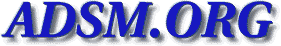Veritas-bu [Top] [All Lists]

## [Veritas-bu] Restore Log Files

2003-07-08 16:53:30
 Subject: [Veritas-bu] Restore Log Files jstephens AT ti DOT com (John D Stephens) Tue, 08 Jul 2003 15:53:30 -0500
 ```Hey - Does anyone know if there is a readable "text" log file of the restore log in NBU 4.5? And if there is, where is it? In 3.4, you could specify the log file before you submitted the job. Is that capability available in 4.5? Thanks, John -- +=+=+=+=+=+=+=+=+=+=+=+=+=+=+=+=+=+=+=+=+ + John D Stephens ITS Design Systems + + Texas Instruments 12500 TI BLVD, Dallas + + jstephens AT ti DOT com 214-480-6229 + +=+=+=+=+=+=+=+=+=+=+=+=+=+=+=+=+=+=+=+=+ ```
 Current Thread [Veritas-bu] Restore Log Files, John D Stephens <= [Veritas-bu] Restore Log Files, tfritz AT cox DOT net [Veritas-bu] Restore Log Files, John D Stephens